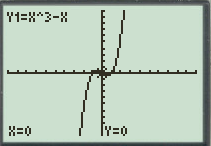# The local maximum and minimum values of the function f ( x ) = x 3 − x and also the values of x on which it occurs.### Precalculus: Mathematics for Calcu...

6th Edition
Stewart + 5 others
Publisher: Cengage Learning
ISBN: 9780840068071### Precalculus: Mathematics for Calcu...

6th Edition
Stewart + 5 others
Publisher: Cengage Learning
ISBN: 9780840068071

#### Solutions

Chapter 3.1, Problem 55E
To determine

## To find: The local maximum and minimum values of the function f(x)=x3−x and also the values of x on which it occurs.

Expert Solution

The local maximum value of the function is equal to 0.38 at x=0.58 and the local minimum value of the function is equal to 0.38 at x=0.58 .

### Explanation of Solution

Given information:

The given function is f(x)=x3x .

Calculation:

Graph the given function f(x)=x3x .

To graph a function y=x3x , follow the steps using graphing calculator.

First press “ON” button on graphical calculator, press Y= key and enter right hand side of the equation y=x3x after the symbol Y1 . Enter the keystrokes X^3X .

The display will show the equation,

Y1=X^3X

Now, press the GRAPH key and TRACE key to produce the graph of given function in standard window as shown in Figure (1).Figure (1)

As observed from the graph, the function has local maximum value equal to 0.38 at x=0.58 .

As observed from the graph, the function has local minima value equal to 0.38 at x=0.58 .

Therefore, the local maximum value of the function is equal to 0.38 at x=0.58 and the local minimum value of the function is equal to 0.38 at x=0.58 .

### Have a homework question?

Subscribe to bartleby learn! Ask subject matter experts 30 homework questions each month. Plus, you’ll have access to millions of step-by-step textbook answers!